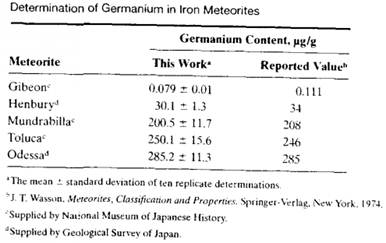### Create an Account

Already have account?

### Forgot Your Password ?

Home / Questions / Nakahara and Wasa determined germanium in meteorites by ICP AES using microwave digestion ...

# Nakahara and Wasa determined germanium in meteorites by ICP AES using microwave digestion and hydride generation 30 Consider the following data table from the referenced paper which compares results

Nakahara and Wasa determined germanium in meteorites by ICP-AES using microwave digestion and hydride generation.30 Consider the following data table from the referenced paper, which compares results of the work with accepted values for germanium in various meteorites.(a) Calculate the 95% confidence intervals for each of the results of Nakahara and Wasa.

(b) Assume that the reported values are the true values for the concentration of germanium in the meteorites, and determine whether the values determined by these workers are equal to or different from the reported values at the 95% confidence level.

(c) Consider the number of significant figures in the standard deviations cited in the table. [s the number of digits justified in each case&#39;) Support your answer with statistical calculations.

Jun 23 2020 View more View Less

#### Answer (Solved)Subscribe To Get Solution